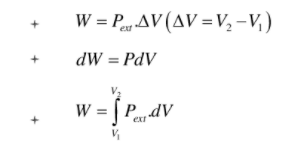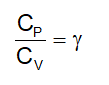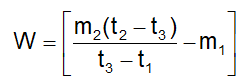# Chemistry formula for class 11 chapter- Thermodynamics

#### Chemistry Formulas

" Thermodynamics" is the sixth chapter of the NCERT Class 11 Chemistry textbook. Thermodynamics is a branch of science that deals with the relationship between heat and other forms of energy. The part of the universe in which the observation is called is a system. The system environment is part of the universe that does not contain a system. Based on the exchange of energy and matter, thermodynamic systems can be divided into three types: closed system, open system, an isolated system.

In a closed system, only energy can be exchanged with the environment. In an open system, power and matter can be exchanged around. In an isolated system, energy and matter cannot be exchanged with the environment.

## Thermodynamics formula

• Universe = system + surrounding
• W = F x dx

Where, W = Work Done

F = Force

Dx= distance moved• Work done by a system = –ve
• Work done on the system = +ve
• C=q/dT; q = Heat absorbed, dT = rise in temperature.
• Q = ΔE+W…………I (law of thermodynamics)
• q = dE + W ……….. I l(aw of thermodynamics)
• Heat absorbed by a system = +ve
• Heat given out by a system = –ve
• H = E + PV
• CP – CV = R, R = Gas constant, CP = Heat capacity at constant pressure
•• Molar heat capacity = specific heat x molecular weight.
• Heat liberating reactions are exothermic reaction, ΔH= –ve
• Heat absorbing reactions are endothermic reaction. ΔH = +ve
• Heat of combustion = Z x θ x M/n, M = molecular weight, m = weight of substance

θ= rise in temperature

Z = heat capacity

• Heat liberated = (W + volume of reaction mixture) x rise in temperaturem1 = mass of water at lower temperature

m2 = mass of water at higher temperature

t01C = Lower temperature

t02C = higher temperature

t03C = resultant temperature

W = water equivalent of calorimeter + stirrer

Do follow NCERT text book and solve questions with the help of Physics Wallah NCERT solutions for class 11 chemsitry